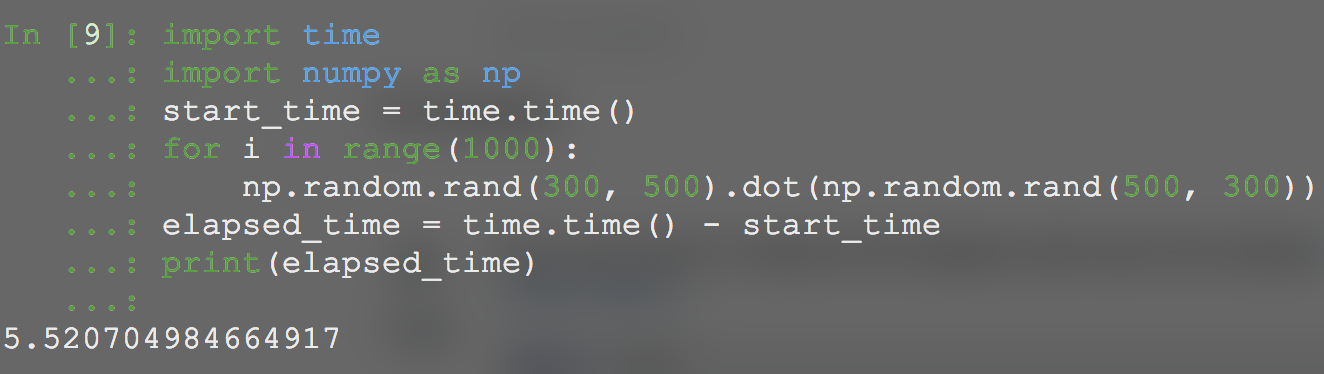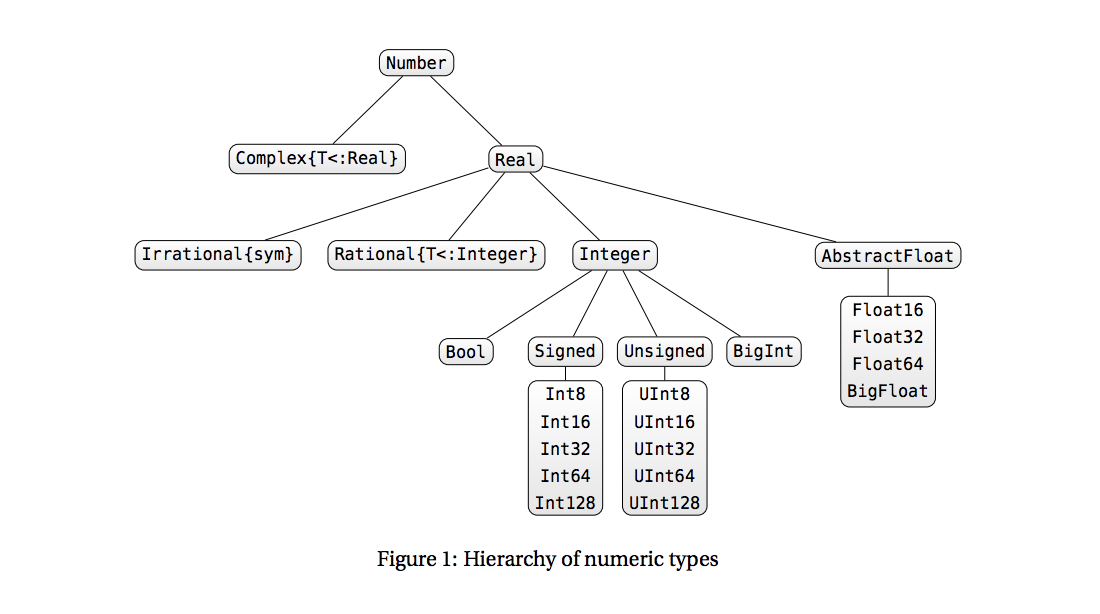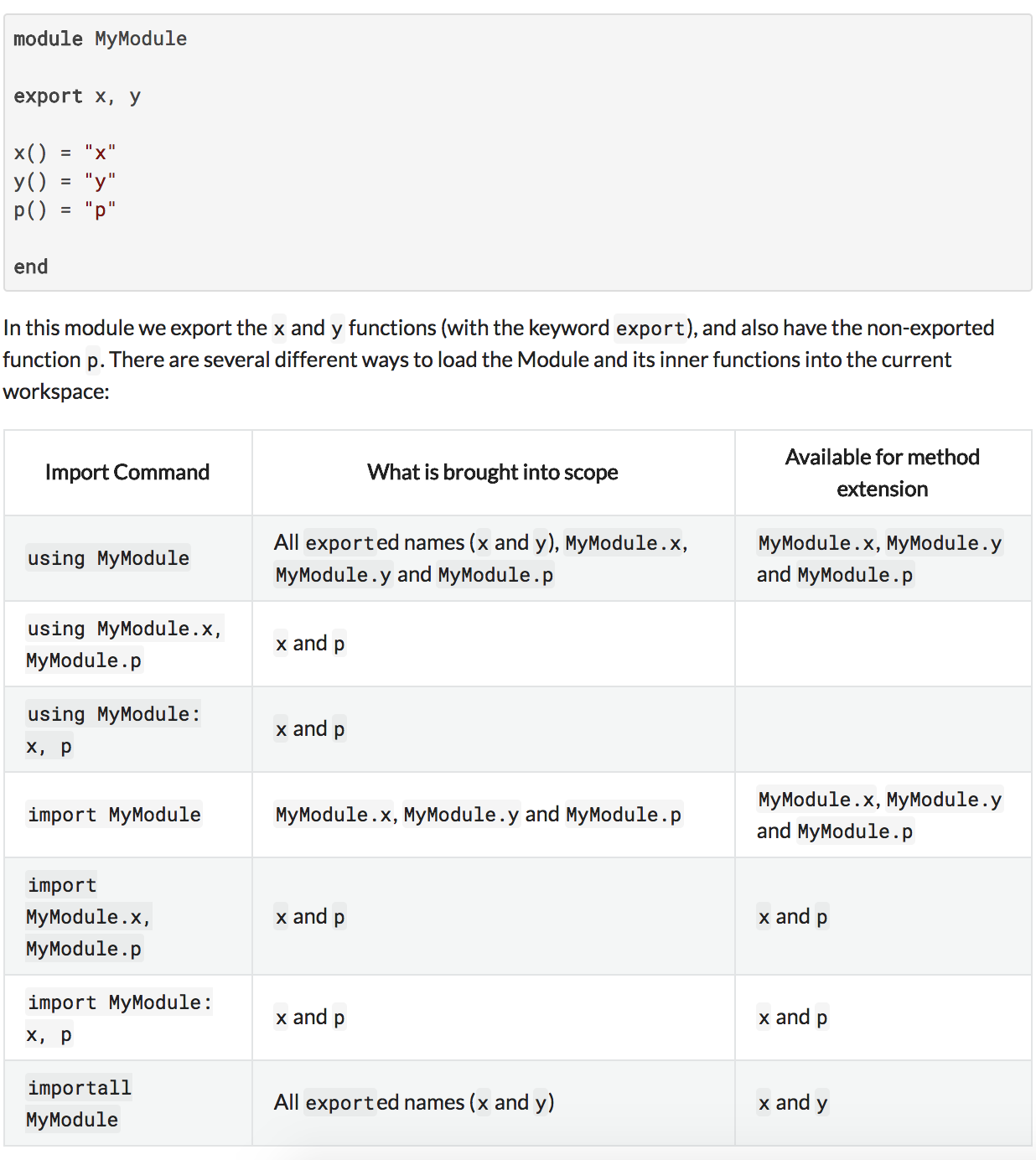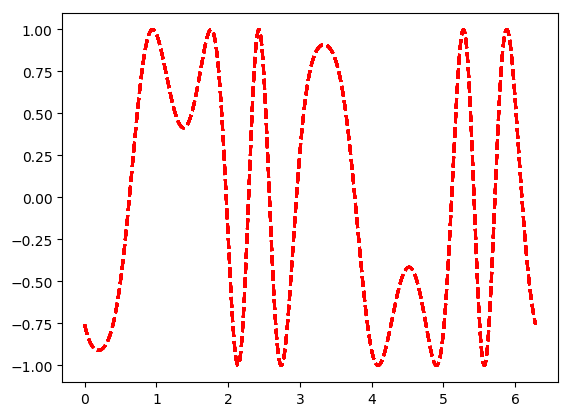### This tutorial is based on Julia 0.6.3

It assumes basic knowledge of programming in other languages, especially in Python and MATLAB, and gives a glance of main features of Julia.

Remember Jupyter Notebook only outputs the last line in a cell if there is no explicit print(stdout).

## Motivation: Calculating Timing

As a motivation, I wrote a basic for loop in Python which performs 1000 matrix multiplications having dimensions of (300, 500).

It took 5.5207 seconds.Now let’s do same thing in Julia. Code is very similar to MATLAB:

tic()
for i= 1:1000
rand(300, 500) * rand(500,300)
end
toc()

Output:
elapsed time: 3.571365137 seconds
3.571365137


It takes 3.5713 seconds. Impressive!

See Julia’s benchmark page for detailed analysis.

## First things first: Print function

It has usual print(without new line), println(with new line), printf(C style formatting) function.

println("a")
print("b")
@printf("%.0f %.1f", 0.5, 0.025)
# NOTE: printf is macro not function, explanation below:
# https://stackoverflow.com/a/19784718/7606396

Output:
a
b1 0.0

# Check version and other stuff
versioninfo()

Output:
Julia Version 0.6.3
Platform Info:
OS: macOS (x86_64-apple-darwin14.5.0)
CPU: Intel(R) Core(TM) i5-6360U CPU @ 2.00GHz
WORD_SIZE: 64
BLAS: libopenblas (USE64BITINT DYNAMIC_ARCH NO_AFFINITY Haswell)
LAPACK: libopenblas64_
LIBM: libopenlibm
LLVM: libLLVM-3.9.1 (ORCJIT, skylake)


### Basic literals and types

1::Int64 # 64-bit integer, no overflow warnings, fails on 32 bit Julia
1.0::Float64 # 64-bit float, defines NaN, -Inf, Inf
true::Bool # boolean, allows "true" and "false"
'c'::Char # character, allows Unicode

# type conversion
Int64(8.0)
Int(8.0) # Prefer this instead of Int64 since it's more generic.
Bool(1)
string(1, true, 13)

Output:
"1true13"

# This gives error
Int64("13")

Output:
MethodError: Cannot convert an object of type String to an object of type Int64
This may have arisen from a call to the constructor Int64(...),
since type constructors fall back to convert methods.

Stacktrace:

 Int64(::String) at ./sysimg.jl:77


convert(String, "13")

Output:
"13"


#### Hierarchy of numeric typesImage Source: http://bogumilkaminski.pl/files/julia_express.pdf

# Returns type
typeof([3.0])

Output:
Array{Float64,1}

# is type of?
isa(1.0, String)

Output:
false

Any # all objects are of this type
Void # type indicating nothing, subtype of Any

Output:
Void

# Tuple
("A", 3/0)
('a', false)::Tuple{Char, Bool}  # Type assertion for tuple

Output:
("A", Inf)


### Arrays, matrices and other beautiful things

# Create an array
reshape(1:12, 3, 4) # 3x4 array filled with 1:12 values
fill("a", 2, 2) # 2x2 array filled with "a"
ones(5) # vector of Float64 ones
a = rand(Float64 ,2, 3, 4) # 2x3x4 array of Chars

Output:
2×3×4 Array{Float64,3}:
[:, :, 1] =
0.945353  0.376008  0.363941
0.96852   0.133192  0.59613

[:, :, 2] =
0.868181  0.791766  0.559305
0.212128  0.990713  0.682902

[:, :, 3] =
0.0322754  0.748374  0.876811
0.429019   0.150571  0.846917

[:, :, 4] =
0.66325    0.459559  0.0278639
0.0417441  0.218397  0.81478

a[1,1,2]

Output:
0.8681809059363892

# Comprehension
[x * y for x in 1:2, y in 1:3] # comprehension generating 2x3 array

Output:
2×3 Array{Int64,2}:
1  2  3
2  4  6

[1, 2]' # Transpose


Output:
1×2 RowVector{Int64,Array{Int64,1}}:
1  2

[1; 2] == [1 2]' # false, different array dimensions
[1, 2, 3] .^ 2 # element wise operations
ndims(a) # number of dimensions in a
eltype(a) # type of elements in a
length(a) # number of elements in a
size(a) # tuple containing dimension sizes of a
vec(a) # cast array to vetor (single dimension)
sum(a, 3) # calculate sums for 3rd dimensions,

# We use -1 for unknown dim in Python.
# Here, ':' is used
# ';' suppress the output as in MATLAB
reshape(a, 3, 2, :);

# ! at the end of function means it changes it's argument.
reverse!([1, 2, 3])

Output:
3-element Array{Int64,1}:
3
2
1

# Accessing is similar to MATLAB
a = reshape(1:12, 3, 4)
a[:, 1:2] # 3x2 matrix
a[:, 1] # 3 element vector
a[1, :] # 4 element vecto

Output:
4-element Array{Int64,1}:
1
4
7
10


### Copy

If the field type is immutable it will be copied by value. If it is mutable, it will be copied by reference.

x = Array{Any}(2)
x = ones(2)
x = trues(3)
a = x
b = copy(x) # shallow copy
c = deepcopy(x) # deep copy
x = "Bang"
x = false
a # identical as x
b # only x changed from
c # same with initial x

Output:
2-element Array{Any,1}:
[1.0, 1.0]
Bool[true, true, true]

# Dictionary
x = Dict("a"=>1)
collect(keys(x))
collect(values(x))
x["a"]

Output:
Base.KeyIterator for a Dict{String,Int64} with 1 entry. Keys:
"a"

"Hello " * "world!" # string concatenation
"Erşan Kuneri " ^ 3 # repeat string

Output:
"Erşan Kuneri Erşan Kuneri Erşan Kuneri "


## Control flows

x = 1 # x is Int32 on 32 bit machine and Int64 on 64 bit machine

Output:
1

# Expression

x = (a = 1; 2 * a) # after: x = 2; a = 1
y = begin
b = 3
3 * b
end # after: y = 9; b = 3

Output:
9

# If statement

if 3 == 2 + 1
z = 1
elseif 1==2
z = 2
else
a = 3
end

# If true A else B
1==2 ? "A" : "B" # standard ternary operator

Output:
"B"

i = 10
while true
i +=1
if i > 10
break
end
end

for x in 1:10
if 3 < x < 5
print(x)
end
end

Output:
4

# Functions
f(x, y=10) = x + y
f(2)

function bloody_function(x::Int, y)
x += 5
# y could be any type
return y
end
bloody_variable = 0
bloody_function(bloody_variable, true)

# Primitives are pass by value
bloody_variable

Output:
0

# MATLAB style function definitions
bloody_function(x::String) = return "Thanks"
# list all methods
methods(bloody_function)

Output:
2 methods for generic function bloody_function:
bloody_function(x::String)
bloody_function(x::Int64, y)

struct Point
x::Int64
y::Float64
meta
end

p = Point(1, 2, "lol")
p.meta

Output:
"lol"

# Use anonymous(lambda?) functions with map
map(x -> x^2 + 2x - 1, [1,3,-1])

Output:
3-element Array{Int64,1}:
2
14
-2

# Multiple values can be returned by constructing tuple
# Parantheses are not must.
function foo(a,b)
a+b, a*b
end

Output:
foo (generic function with 1 method)

# Functions with keyword arguments are defined using a semicolon
# in the signature:

function plot_it(x, y; style="solid", width=1, color="black")
###some plotting code here
end

Output:
plot_it (generic function with 1 method)

[1 2] .< [2 1] # vectorized operators need ’.’
# multiplication can be omitted
2x + 2(x+1)

Output:
42

[1 2 3] # 1×3 Array{Int64,2}:
[1, 2, 3] # 3-element Array{Int64,1}:

Output:
3-element Array{Int64,1}:
1
2
3

# broadcasting is possible but a bit different from Numpy
x = [1 2 3]
y = [1, 2, 3]
z = reshape(1:9, 3, 3)
#z + x # error
z .+ x # x broadcasted vertically
z .+ y # y broadcasted horizontally

Output:
3×3 Array{Int64,2}:
2  5   8
4  7  10
6  9  12


### Types

Julia’s type system is dynamic, but gains some of the advantages of static type systems by making it possible to indicate that certain values are of specific types.

Many Julia programmers may never feel the need to write code that explicitly uses types. Some kinds of programming, however, become clearer, simpler, faster and more robust with declared types.

The :: operator can be used to attach type annotations to expressions and variables in programs. 2 reasons to do this:

• To provide extra type information to the compiler, which can then improve performance in some cases

• As an assertion to help confirm that your program works the way you expect,

### Modules## IO operations

# Write
f = open("dummy.txt", "w+")
write(f, "Quite Pythonic...\nRight?")
close(f)

open("dummy.txt") do f
for l in eachline(f)
println(l)
end
end

Output:
Quite Pythonic...
Right?


## Plotting

Plotting in Julia is available through external packages. Please check official documentation.

x = linspace(0,2*pi,1000); y = sin.(3*x + 4*cos.(2*x))
plot(x, y, color="red", linewidth=2.0, linestyle="--")?workspace()
# Above calls help for given function
# See workspace's description.

workspace()

Output:
Replace the top-level module (Main) with a new one, providing a clean workspace. The previous Main module is made available as LastMain. A previously-loaded package can be accessed using a statement such as using LastMain.Package.

This function should only be used interactively.